# Design of highly reflective film for smart radiation device

## Xiaoqian Wang1, Junlin Yang2, Donglai Li3, Hui Ye4, Chen Ling5, Jingbo Li6, Haibo Jin7

1, 2, 3, 5, 6, 7Beijing Key Laboratory of Construction Tailorable Advanced Functional Materials and Green Applications, Beijing Institute of Technology, Beijing, 100081, China

4Aerospace Research Institute of Materials and Processing Technology, Beijing, 100076, China

4Corresponding author

Vibroengineering PROCEDIA, Vol. 40, 2022, p. 132-138. https://doi.org/10.21595/vp.2022.22385
Received 4 January 2022; received in revised form 17 January 2022; accepted 25 January 2022; published 5 February 2022

54th International Conference on Vibroengineering, Shanghai, China, February 5, 2022

Copyright © 2022 Xiaoqian Wang, et al. This is an open access article distributed under the Creative Commons Attribution License, which permits unrestricted use, distribution, and reproduction in any medium, provided the original work is properly cited.
ERRATUM. The corresponding author numbers were set incorrectly in the paper finally approved (after the acceptance) by the authors. For more information read Editor's Note.
Views 134
CrossRef Citations 0
Abstract.

Smart radiation device (SRD) based on the asymmetrical Fabry-Perot cavity automatically tune its IR emittance depending on the ambient temperature, making it an ideal choice for thermal control system of spacecrafts. The low solar absorption is desirable for SRD to prevent the spacecraft from overheating by sun light. In this paper, a multilayer highly reflective film with ${\left(LH\right)}^{k}$ stacking layers is designed to reduce the solar absorptance (${A}_{s}$) of the Ag/Al2O3/VO2 structured SRD. The reflective film achieves a high reflection band in ~220 nm bandwidth from 460 nm to 680 nm, which results in a reduction of the solar absorptance by 25.56 % for SRD working at low temperature and 24.27 % at high temperature as the stacking factor 5. The simulation results indicate that an economic reflective film with 3 can achieve effective suppression of ${A}_{s}$ of SRD, demonstrating the promising potential of the proposed reflective film in thermal control application of spacecrafts.

Highlights
• The reflective film achieves a high reflection band in ~220 nm bandwidth from 460 nm to 680 nm.
• The (LH)^k stacking layers with k=5 performed the most effective reduction of As by 25.56% as SRD works at high temperature and 24.27% at low temperature.
• The structure has simple process and low cost, and can be used for large-area production.

Keywords: smart radiation device (SRD), Fabry-Perot cavity, highly reflective film, solar absorption, emittance.

#### 1. Introduction

In space environment, radiation is the only way to exchange heat between spacecraft and external space . The emittance devices, a key part of the thermal control system, are designed to radiate heat produced by electronic systems. The variable emittance devices have been attracting extensive attention because of its bi-functionality that achieves heat preservation at low-temperature and heat dissipation at high-temperature . The smart radiation device (SRD) based on thermochromic materials can automatically adjust the emittance depending on temperature, achieving low-emittance at low temperature and high-emittance at high temperature . Furthermore, the SRD does not need accessories as the traditional variable emittance devices, such as motorized shutters, which require components involving thermal sensors, actuators, power supplies and circuit systems, resulting in the addition of load and volume to the spacecraft as well as the risk of damage. Thus, the SRD is a promising technique opening up horizon for satellite thermal control.

Benkahoul et al. reported the first fabrication of the VO2-based SRD by depositing VO2 film on the strongly reflective Al substrate, taking use of the thermochromic property of VO2 in infrared region . VO2 undergoes a metal-insulator transition at ~68 ℃ (${T}_{c}$), [5-8] and is transparent to visible and infrared (IR) light as $T<{T}_{c}$, and becomes strong IR reflective as $T>{T}_{c}$, leading to the IR transmittance modulation more than 70 % [9, 10]. Hendaoui et al.  designed and fabricated Au/SiO2/VO2 structure devices to achieve an intense high-temperature emittance (${\epsilon }_{H}$) of 0.8 and a large emittance modulation ($∆\epsilon$) of 0.46. Wang et al. reported a Ag/HfO2/VO2 three-layer SRD which realized a $∆\epsilon$ of 0.55 . Beaini et al. studied the effect of individual layers on the SRD performance, and achieved a large $∆\epsilon =$ 0.66 by selecting BaF2 as the optical medium layer . However, those works did not involve the investigation on solar absorption (${A}_{s}$).

In space environment, the sun light can heat the spacecraft surface up to ~150 ℃, the low solar absorption rate is essential for the thermal control surface to avoid overheating of solar radiation. Therefore, a low solar absorption SRD is desirable for the integral thermal control system of microsatellites. The surface micro- and nano- optical structure can realize the low solar absorption surface [14, 15]. However, the micro- and nano-processing suffers from harsh experimental techniques and difficulty of preparing large area surface. In this paper, we propose an ideal of depositing reflective film on the surface of SRD to achieve the low solar absorption, and do not damage the IR emittance of the devices. The reflective film is designed to alternatively stack low refractive index layer ($L$, Al2O3) and high refractive index layer ($H$, TiO2) on the Ag/Al2O3/VO2 device. The optical simulation demonstrates that the optical design reduces the ${A}_{s}$ by ~25 % while slightly improves the $∆\epsilon$ by the ${\left(LH\right)}^{k}$ stacking with 5.

#### 2.1. SRD structure

As shown in Fig. 1, the SRD structure is composed of VO2 film (50 nm), optical medium Al2O3 film (1500 nm) and highly reflecting Ag film (200 nm). Optical constants of VO2 film in cold and hot states are from . At low temperature, VO2 is a semiconductor, transparent to the IR light, so the IR radiation can easily pass through the VO2 and Al2O3 layers and reflected by the Ag layer. At high temperature, VO2 works as an IR reflector, together with Al2O3 layer and Ag layer to form an asymmetric Fabry-Perot cavity (F-P cavity), which can realize interference extinction for IR light around 10 μm, resulting in high IR emittance.

Fig. 1. Illustration of structure and tunable emittance of SRD, a) for insulating VO2 at low temperatures, b) metallic VO2 at high temperatures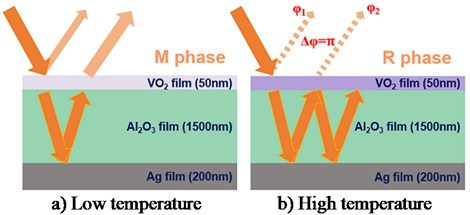According to Kirchhoff’s law, the optical absorptance ($\alpha$) of an object is equivalent to its emittance ($\epsilon$) under thermal equilibrium conditions, namely $\epsilon \left(\lambda \right)=\alpha \left(\lambda \right)$. The IR emittance at a certain temperature ${\epsilon }_{T}\left(\lambda \right)$ is an integral value calculated using Eq. (1) :

(1)
${\epsilon }_{IR}\left(\lambda \right)=\frac{{\int }_{{\lambda }_{1}}^{{\lambda }_{2}}{\alpha }_{IR}\left(\lambda \right){B}_{IR}\left(\lambda \right)d\lambda }{{\int }_{{\lambda }_{1}}^{{\lambda }_{2}}{B}_{IR}\left(\lambda \right)\mathrm{d}\lambda },$

where ${B}_{IR}\left(\lambda \right)$ is the blackbody spectral radiation at the desired temperature given by Planck’ law.

#### 2.2. Reflective film

The highly reflective film is fabricated by alternatively stacking of high refractive layer and low refractive layer, whose optical thickness $nh$ ($n$ denotes the refractive index) is ${\lambda }_{0}$/4. As shown in Fig. 2, the high refractive index material is TiO2 (2.38 at 550 nm) and the low refractive index material is Al2O3 (1.62 at 550 nm).

Fig. 2. Schematic of highly reflective layer structure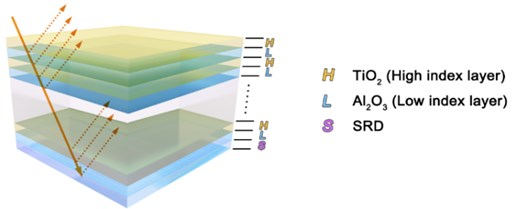The model structure can be represented by the following expression:

(2)
$GLHLHLH...LHA=G\left(LH{\right)}^{k}A.$

$G$ denotes substrate, in this case, it is SRD device; $A$ is air; $L$ and $H$ represent the low refractive layer and high refractive layer, respectively; $k$ is the stacking factor, and 1 means a couple of $L$ and $H$ layers.

For a single-layer optical film with ${\lambda }_{0}$/4, the reflectance is:

(3)
$R={\left(\frac{{n}_{0}-{n}_{1}^{2}/{n}_{2}}{{n}_{0}+{n}_{1}^{2}/{n}_{2}}\right)}^{2},$

where ${n}_{0}$ is the refractive of air, ${n}_{1}$ is the refractive of optical film, ${n}_{2}$ is the refractive of substrate. Eq. (3) can be expressed as Eq. (4) by taking ${n}_{\mathrm{I}}={n}_{1}^{2}/{n}_{2}$:

(4)
$R={\left(\frac{{n}_{0}-{n}_{Ⅰ}}{{n}_{0}+{n}_{I}}\right)}^{2},$

where ${n}_{I}$ is defined as the equivalent refractive index of the optical structure constructed by the ${\lambda }_{0}$/4 ${n}_{1}$ layer covering the ${n}_{2}$ substrate, namely the ${n}_{1}/{n}_{2}$ structure is equivalent to a ${n}_{I}$ medium. In this way, the equivalent refractive index ${n}_{2k}$ of the ${\left(LH\right)}^{k}$ stacking film is:

(5)
${n}_{2k}={\left(\frac{{{n}_{H}}^{2}}{{{n}_{L}}^{2}}\right)}^{k}{n}_{2},$

and the reflectivity of the (LH)k film becomes:

(6)
${R}_{2k}={\left(\frac{1-{n}_{0}/{n}_{2k}}{1+{n}_{0}/{n}_{2k}}\right)}^{2}.$

As $k$ increases, ${n}_{2k}$ becomes much larger than ${n}_{0}$ according to Eq. (5), then ${R}_{2k}$ tends to be 1. The highly reflective films are obtained.

For SRD, the strong reflecting Ag layer determines the transmittance $T\left(\lambda \right)$ of SRD is 0, then $\alpha \left(\lambda \right)=1-R\left(\lambda \right)$ according to $\alpha \left(\lambda \right)+R\left(\lambda \right)+T\left(\lambda \right)=1$ . The solar absorption ${A}_{s}$ of the devices can be calculated using the equation:

(7)
${{A}_{s}}_{-\mathrm{s}\mathrm{o}\mathrm{l}}=\frac{{\int }_{{\lambda }_{1}}^{{\lambda }_{2}}\left(1-{R}_{sol}\left(\lambda \right)\right){\phi }_{sol}d\lambda }{{\int }_{{\lambda }_{1}}^{{\lambda }_{2}}{\phi }_{sol}d\lambda },$

where ${\phi }_{sol}$ represents the solar radiation spectrum corresponding to an atmospheric mass of 1.5 (according to the sun standing 37° above the horizon).

#### 3. Results and discussion

Optical simulation results of the VO2/Al2O3/Ag three-layer SRD are shown in Fig. 3. In the visible region, the SRD working at low temperature and high temperature has the similar reflectance value as shown in Fig. 3(a). However, in the NIR (800-3000 nm) region, the reflectance at low temperature is obviously higher than that at high temperature. This is because the M-phase VO2 is transparent to both visible and IR light but the R-phase VO2 is highly reflective to IR light while retains transparent to visible light. The calculated solar absorptance ${A}_{s}$ of the SRD is 33.29 % at low temperature and 45.52 % at high temperature, as shown in Fig. 3(c). The infrared emittance spectra of the SRD is shown in Fig. 3(d). The device achieves the high emittance at high temperature and low emittance at low temperature. The emittance modulation $\mathrm{\Delta }\epsilon$ is up to 0.71 with 0.12 and 0.83, indicating the Fabry-Perot cavity works well. However, the solar absorptance of the device is unsatisfactory due to the high values in Fig. 3(a-c), especially for that of high temperature.

Fig. 3. Simulation results of VO2/Al2O3/Ag three-layer structured SRD: a) UV-VIS and b) NIR reflection spectra of M- and R-phase VO2; c) Integral solar absorptance ${A}_{s}$ of M- and R-phase VO2; d) The emittance spectra of M- and R-phase VO2. The $\epsilon$ values in d) are the integral emittances (5-15 μm) of $M$ and $R$ phases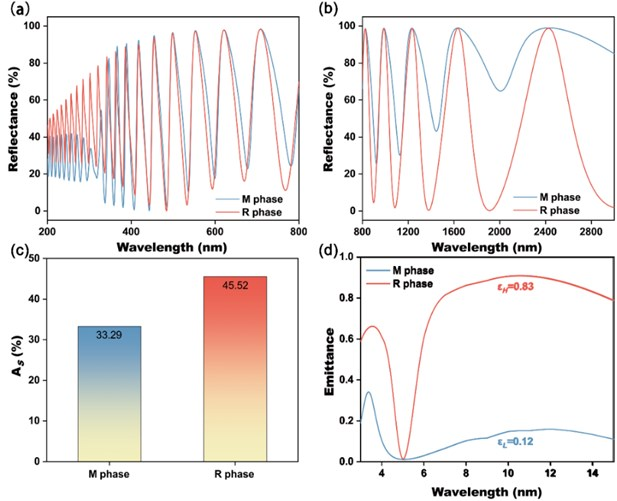Fig. 4(a) shows the calculated reflection spectra of the reflective films with $k$ changing from 1 to 6. The effective high-reflection bandwidth of the reflective films becomes narrow with the increase of $k$, and the peak value of the strong reflection band increases with $k$. For the reflective film of $k=$ 6, the peak value of the strong reflection band reaches 99 %, and the effective high-reflection bandwidth is about 220 nm from 460 nm to 680 nm. Fig. 4(b) shows the integral reflectance of the reflective films in the wavelength range of 300-800 nm. The integral reflectance increases with $k$, and reaches the largest value when 5. In fact, the integral reflectance does change a lot as $k\ge$ 4.

Fig. 4. a) Calculated reflection spectra of the reflective films with different stacking factors $k$, b) integral reflectance in the 300-800 nm range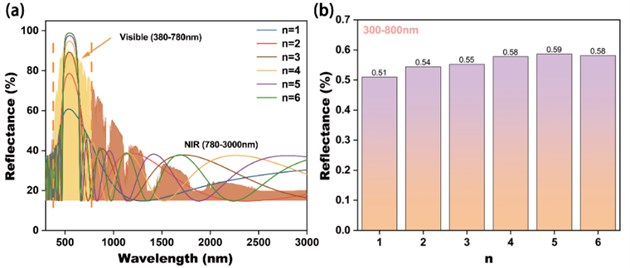Fig. 5 shows the calculated reflection spectra of the highly reflective film coated SRD in the solar spectral range. The highly reflective coating renders SRD the quite high reflectance in the wavelength range of 460-680 nm, and has less impact on other bands. The solar spectral reflectance of SRD rises up with the increase of stacking factor k. The integral solar absorptances of the devices are calculated and listed in Table 1. As 5, the reflective film coated SRD has the lowest ${A}_{s}$, 24.78 % for low-temperature (M-phase VO2) and 34.47 % for high-temperature (R-phase VO2), corresponding to a reduction of 25.56 % and 24.27 % with respect to the bared SRD, respectively. Considering economy and feasibility, the 3 film can also do good work on reducing the solar absorption of SRD as shown in Table 1.

Table 1. Calculated integral solar absorptance ${A}_{s}$ of SRD with highly reflective films

 VO2 $k$ 0 1 2 3 4 5 6 M phase 33.29 % 30.96 % 27.12 % 26.64 % 25.19 % 24.78 % 25.51 % R phase 45.52 % 40.06 % 36.27 % 34.71 % 35.5 % 34.47 % 36.12 %

Fig. 5. Simulated solar reflection spectra of SRD devices with highly reflective films, a), b) for M-phase VO2, c), d) R-phase VO2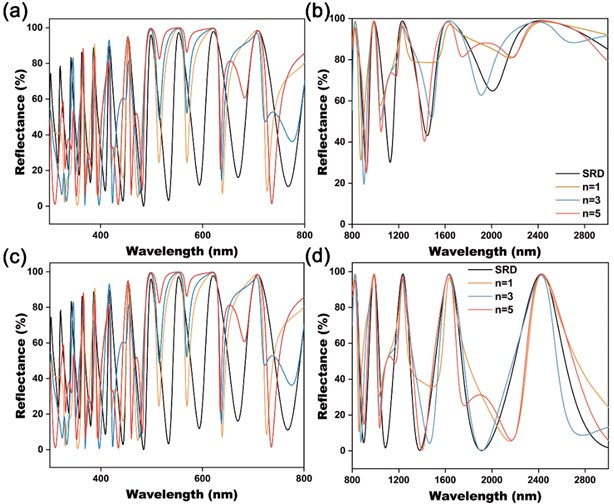The IR emittance of the highly reflective film coated SRD is calculated to find out whether the addition of the reflective film injures the emittance performance of SRD. Fig. 6 shows the calculated emittance spectra of SRD with highly reflective films. The IR emittance modulation $∆\epsilon$ of SRD is enhanced by increasing $k$. What it is that the emittance of SRD at high temperature is increased and decreased at low temperature in the 5-16 μm range with the increase of $k$. The improvement of $∆\epsilon$ is mainly ascribed to the broadening and enhancing of the IR absorption band at high temperature as shown in Fig. 6.

Fig. 6. The emittance spectra of SRD devices with highly reflective film for VO2 in M/R phase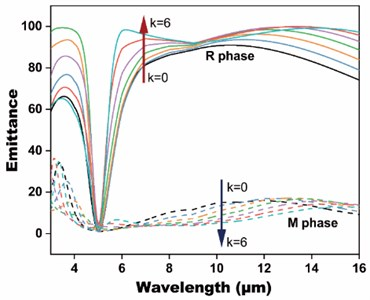#### 4. Conclusions

The highly reflective film was designed with the classical ${\left(LH\right)}^{k}$ stacking model to reduce the solar absorptance of SRD. The ${\left(LH\right)}^{k}$ stacking layers with 5 performed the most effective reduction of ${A}_{s}$ by 25.56 % as SRD works at high temperature and 24.27 % at low temperature. Meanwhile, the highly reflective film can enhance the high-temperature emittance and suppress the low-temperature emittance in the 5-16 μm range, as a result, improve the IR emittance modulation of SRD. In the view of economy, the reflective film of 3 can bring about the effective reduction of the solar absorptance of SRD, making the proposed highly reflective film feasible.

#### Acknowledgements

This work was supported by the National Natural Science Foundation of China (Grant Nos. 51972029 and 51572027).

1. A. L. Alexander, “Thermal control in space vehicles,” Science, Vol. 143, No. 3607, pp. 654–660, Feb. 1964, https://doi.org/10.1126/science.143.3607.654 [Publisher]
2. H. Jin, C. Ling, and L. I. Jingbo, “Development of variable-emittance thermal control technology,” Journal of Deep Space Exploration, 2018. [Search CrossRef]
3. M. Benkahoul et al., “Thermochromic VO2 film deposited on Al with tunable thermal emissivity for space applications,” Solar Energy Materials and Solar Cells, Vol. 95, No. 12, pp. 3504–3508, Dec. 2011, https://doi.org/10.1016/j.solmat.2011.08.014 [Publisher]
4. D. H. Ren and J. F. Tian, “Modeling and analysis of a MEMS-based thermal emissivity variable thermal control device,” in Applied Mechanics and Materials, Vol. 401-403, pp. 1686–1690, Sep. 2013, https://doi.org/10.4028/www.scientific.net/amm.401-403.1686 [Publisher]
5. J. B. Goodenough, “2 components of crystallographic transition in VO2,” Journal of Solid State Chemistry, Vol. 3, No. 4, p. 490, 1971. [Publisher]
6. J. Cao et al., “Extended Mapping and exploration of the vanadium dioxide stress-temperature phase diagram,” Nano Letters, Vol. 10, No. 7, pp. 2667–2673, Jul. 2010, https://doi.org/10.1021/nl101457k [Publisher]
7. J. Zhang et al., “Self-assembling VO2 nanonet with high switching performance at wafer-scale,” Chemistry of Materials, Vol. 27, No. 21, pp. 7419–7424, Nov. 2015, https://doi.org/10.1021/acs.chemmater.5b03314 [Publisher]
8. Y. Cui et al., “Thermochromic VO2 for energy-efficient smart windows,” Joule, Vol. 2, No. 9, pp. 1707–1746, Sep. 2018, https://doi.org/10.1016/j.joule.2018.06.018 [Publisher]
9. F. Guinneton, L. Sauques, J.-C. Valmalette, F. Cros, and J.-R. Gavarri, “Role of surface defects and microstructure in infrared optical properties of thermochromic VO2 materials,” Journal of Physics and Chemistry of Solids, Vol. 66, No. 1, pp. 63–73, Jan. 2005, https://doi.org/10.1016/j.jpcs.2004.08.032 [Publisher]
10. Z. Zhao et al., “Sn-W co-doping improves thermochromic performance of VO2 films for smart windows,” ACS Applied Energy Materials, Vol. 3, No. 10, pp. 9972–9979, Oct. 2020, https://doi.org/10.1021/acsaem.0c01651 [Publisher]
11. A. Hendaoui, N. Émond, S. Dorval, M. Chaker, and E. Haddad, “VO2-based smart coatings with improved emittance-switching properties for an energy-efficient near room-temperature thermal control of spacecrafts,” Solar Energy Materials and Solar Cells, Vol. 117, pp. 494–498, Oct. 2013, https://doi.org/10.1016/j.solmat.2013.07.023 [Publisher]
12. X. Wang, Y. Cao, Y. Zhang, L. Yan, and Y. Li, “Fabrication of VO2-based multilayer structure with variable emittance,” Applied Surface Science, Vol. 344, pp. 230–235, Jul. 2015, https://doi.org/10.1016/j.apsusc.2015.03.116 [Publisher]
13. R. Beaini, B. Baloukas, S. Loquai, J. E. Klemberg-Sapieha, and L. Martinu, “Thermochromic VO2-based smart radiator devices with ultralow refractive index cavities for increased performance,” Solar Energy Materials and Solar Cells, Vol. 205, p. 110260, Feb. 2020, https://doi.org/10.1016/j.solmat.2019.110260 [Publisher]
14. K. Tang et al., “Temperature-adaptive radiative coating for all-season household thermal regulation,” Science, Vol. 374, No. 6574, pp. 1504–1509, Dec. 2021, https://doi.org/10.1126/science.abf7136 [Publisher]
15. K. Sun et al., “VO 2 thermochromic metamaterial-based smart optical solar reflector,” ACS Photonics, Vol. 5, No. 6, pp. 2280–2286, Jun. 2018, https://doi.org/10.1021/acsphotonics.8b00119 [Publisher]
16. Y. Ge, F. Zhao, L. Wang, and X. Wang, “A novel perspective on the design of thermochromic VO2 films: combining ab initio calculations with FDTD simulations,” Surface and Coatings Technology, Vol. 402, p. 126493, Nov. 2020, https://doi.org/10.1016/j.surfcoat.2020.126493 [Publisher]
17. J. R. Howell, R. Siegel, and M. P. Mengüç, “Thermal radiation heat transfer,” National Aeronautics and Space Administration, 1969. [Search CrossRef]